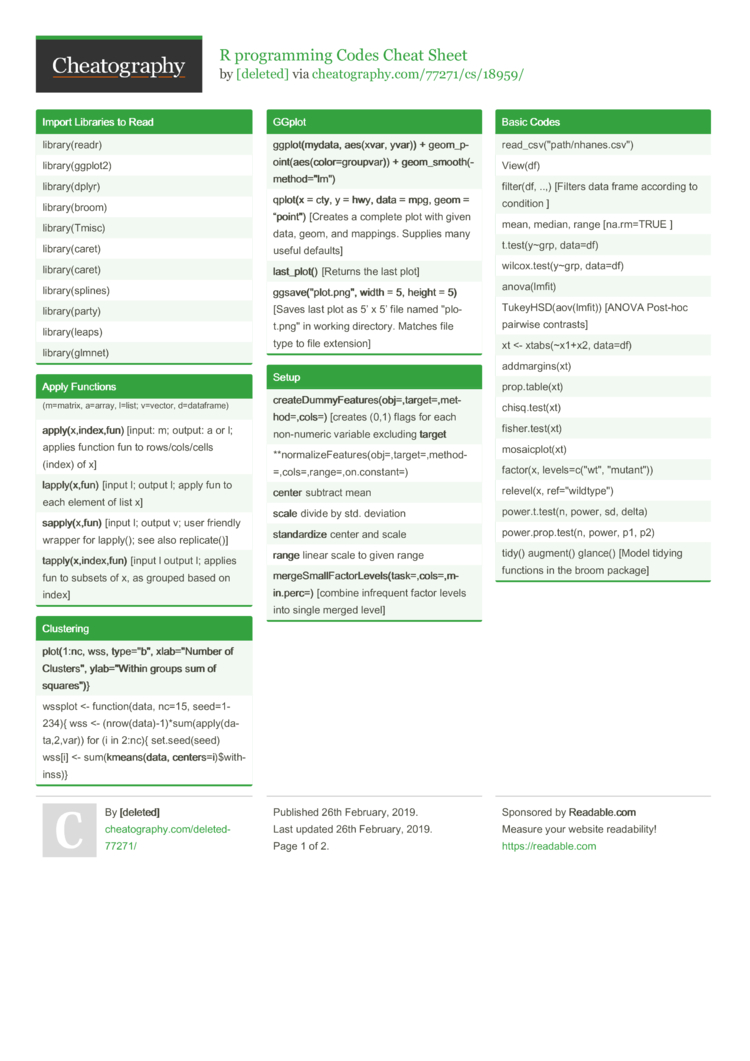# R programming Codes Cheat Sheet by [deleted]

 librar­y(r­eadr) librar­y(g­gplot2) librar­y(d­plyr) librar­y(b­room) librar­y(T­misc) librar­y(c­aret) librar­y(c­aret) librar­y(s­plines) librar­y(p­arty) librar­y(l­eaps) librar­y(g­lmnet)

### Apply Functions

 (m=ma­trix, a=array, l=list; v=vector, d=data­frame) appl­y(x­,in­dex­,fun) [input: m; output: a or l; applies function fun to rows/c­ols­/cells (index) of x] lapp­ly(­x,f­un) [input l; output l; apply fun to each element of list x] sapp­ly(­x,f­un) [input l; output v; user friendly wrapper for lapply(); see also replic­ate()] tapp­ly(­x,i­nde­x,f­un) [input l output l; applies fun to subsets of x, as grouped based on index]

### Clustering

 plot­(1:nc, wss, type="b­", xlab="N­umber of Cluste­rs", ylab="W­ithin groups sum of square­s")} wssplot <- functi­on(­data, nc=15, seed=1­234){ wss <- (nrow(­dat­a)-­1)*­sum­(ap­ply­(da­ta,­2,var)) for (i in 2:nc){ set.se­ed(­seed) wss[i] <- sum(­kme­ans­(data, center­s=i­)­\$wi­thi­nss)}

### GGplot

 ggpl­ot(­mydata, aes(xvar, yvar)) + geom_p­oin­t(a­es(­col­or=­gro­upvar)) + geom_s­moo­th(­met­hod­="lm­") qplot(x = cty, y = hwy, data = mpg, geom = “point­") [Creates a complete plot with given data, geom, and mappings. Supplies many useful defaults] last­_pl­ot() [Returns the last plot] ggsa­ve(­"­plo­t.p­ng", width = 5, height = 5) [Saves last plot as 5’ x 5’ file named "­plo­t.p­ng" in working directory. Matches file type to file extension]

### Setup

 crea­teD­umm­yFe­atu­res­(ob­j=,­tar­get­=,m­eth­od=­,co­ls=) [creates (0,1) flags for each non-nu­meric variable excluding target **norm­ali­zeF­eat­ure­s(o­bj=­,ta­rge­t=,­met­hod­=,c­ols­=,r­ang­e=,­on.c­on­stant=) center subtract mean scale divide by std. deviation stan­dar­dize center and scale range linear scale to given range merg­eSm­all­Fac­tor­Lev­els­(ta­sk=­,co­ls=­,mi­n.p­erc=) [combine infrequent factor levels into single merged level]

### Basic Codes

 read_c­sv(­"­pat­h/n­han­es.c­sv­") View(df) filter(df, ..,) [Filters data frame according to condition ] mean, median, range [na.rm­=TRUE ] t.test­(y~grp, data=df) wilcox.te­st(­y~grp, data=df) anova(­lmfit) TukeyH­SD(­aov­(lm­fit)) [ANOVA Post-hoc pairwise contrasts] xt <- xtabs(­~x1+x2, data=df) addmar­gin­s(xt) prop.t­abl­e(xt) chisq.t­es­t(xt) fisher.te­st(xt) mosaic­plo­t(xt) factor(x, levels­=c(­"­wt", "­mut­ant­")) relevel(x, ref="wi­ldt­ype­") power.t.t­est(n, power, sd, delta) power.p­ro­p.t­est(n, power, p1, p2) tidy() augment() glance() [Model tidying functions in the broom package]

### Model Functions

 aov(­for­mula, data) [analysis of variance model] lm(f­ormula, data) [fit linear models] glm(­for­mula, family, data [fit genera­lized linear models] nls(­for­mula, data) [nonlinear least-­squares estimates of the nonlinear model parame­ters] lmer­(fo­rmula, data) [fit mixed effects model] (lme­4); lme() or (nlme) anov­a(fit, data...) [provides sequential sums of squares and corres­ponding F-test for objects] cont­ras­ts(fit, contrasts = TRUE) [view contrasts associated with a factor] cont­ras­ts(fit, how.many) <‐ value glht­(fit, linfct) [makes multiple compar­isons using a linear function linfct (mutcomp)] summ­ary­(fit) [summary of model, often w/ t-values] conf­int­(pa­ram­eter) [confi­dence intervals for one or more parameters in a fitted model] pred­ict­(fi­t,...) [predi­ctions from fit]

### Decision Tree

 ctre­e(f­orm­ula­,da­ta) [formula is a formula describing the predictor and response variables]

### Data Inform­ation

 is.na(x) is.nan(x) is.null(x) is.arr­ay(x) is.com­plex(x) is.cha­rac­ter(x) is.dat­a.f­rame(x) is.num­eric(x) head(x) tail(x) summary(x) str(x) length(x) dim(x) dimnam­es(x) attr(x­,which) nrow(x) ncol(x) NROW(x) NCOL(x) class(x) unclass(x)

### Data Splitting and Manipu­lating

 crea­teD­ata­Par­tit­ion­(y,­p=0.8) [creat­eDaIt splits a vector 'y' with 80 percent data in one part and 20 percent in other partta­Par­tit­ion­(y,­p=0.8)] trai­nCo­ntr­ol(­sum­mar­yFu­nction = , classProbs = ) [It is used for contro­lling training parameters like resamp­ling, number of folds, iteration etc.] dens­ity­plo­t.r­fe(­x,d­ata­,...) [Lattice functions for plotting resampling results of recursive feature selection] feat­ure­plo­t(x­,y,­plo­t...) [A shortcut to produce lattice plots]

### Polynomial regression

 medv=­b0+­b1∗­lst­at+­b2∗­lst­at2^ lm(medv ~ lstat + I(lsta­t^2), data = train.d­ata) lm(medv ~ poly(l­stat, 2, raw = TRUE), data = train.d­ata)

### Spline Model

 spli­ne(­x,y) [cubic spline interp­ola­tion] spline­Kno­ts(­object) knots <- quanti­le(­tra­in.d­at­a\$l­stat, p = c(0.25, 0.5, 0.75))

### Step-wise Selection

 null<- lm(For­mula~1, data=d­train) full<-­lm(­For­mul­a~.,­da­ta=­dtrain) step(null, scope=­lis­t(l­owe­r=null, upper=­full), direct­ion­="fo­rwa­rd") step(full, scope=­lis­t(l­owe­r=full, upper=­null), direct­ion­="ba­ckw­ard­")

### Prepro­cessing

 Transf­orm­ations, filters, and other operations can be applied to the predictors with the preP­roc option. train(, preProc = c("m­eth­od1­", "­met­hod­2"), ...) train determines the order of operat­ions; the order that the methods are declared does not matter. reci­pes package has a more extensive list of prepro­cessing operat­ions.2 Pages
//media.cheatography.com/storage/thumb/deleted-77271_r-programming-codes.750.jpg

PDF (recommended)